#The Wolfram Language:Fast Introduction for Programmers

All sections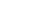Get Started

# Function DefinitionsVideo Version

In the Wolfram Language, function definitions are just assignments that give transformation rules for patterns.

Notes for Java programmers:

Unlike methods in the object-oriented Java language, Wolfram Language functions are not restricted to a class hierarchy.

Define a function of two arguments named x and y:

In:=1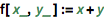`f[x_, y_] := x + y`

Notes for Java programmers:

In basic Wolfram Language function definitions, parameter names are followed by _ ("blank"). Unlike Java methods, Wolfram Language functions will accept any type of data by default.

Notes for Python programmers:

In basic Wolfram Language function definitions, parameter names are followed by _ ("blank"), allowing parameters to correspond to arbitrary symbolic patterns. This enables powerful and flexible function definitions not possible by default in Python. You can define Wolfram Language–based functions for use in Python using session.function from the Wolfram Client Library for Python.

Use the definition:

In:=2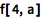`f[4, a]`
Out=2Clear the definition:

In:=3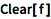`Clear[f]`

Functions can be defined by specifying their values in a sequence of cases:

In:=1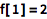`f = 2`
Out=1In:=2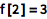`f = 3`
Out=2Any undefined case is left untransformed:

In:=3`{f, f, f, f}`
Out=3You can use /; to restrict a definition to apply only when a particular condition holds:

In:=4`f[x_, y_] := x - y /; x > y`

Notes for Java programmers:

Using /; in the Wolfram Language is like a shortened If statement.

Notes for Python programmers:

Using /; in the Wolfram Language is like an if statement that can be applied to a function, allowing a function to flexibly choose a definition based on a condition.

You can define transformations for any left-hand side—a convenient way to "destructure" arguments:

In:=1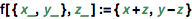`f[{x_, y_}, z_] := {x + z, y - z}`

In:=2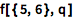`f[{5, 6}, q]`
Out=2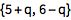The Wolfram Language doesn't have types as such; everything is a symbolic expression. The "head" of an expression can be used as a kind of generalized type designator.

Give different definitions for what f should do when applied to a "u object" or a "v object":

In:=1`f[u[x_]] := x + 1`

In:=2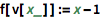`f[v[x_]] := x - 1`

Notes for Java programmers:

This is similar to overloading a Java method for different data types, except that Wolfram Language functions can be overloaded more generally, based on the symbolic structure of their arguments.

Notes for Python programmers:

A Wolfram Language function can use built-in pattern matching to change its behavior based on the symbolic structure of any of its arguments. Achieving this in Python would require fairly elaborate custom code or third-party libraries.

QUICK REFERENCE: Defining Variables and Functions

Which of the following defines a function f that adds two numbers (e.g., f[3, 4] == 7)?

Which definition would make {f, f, f, f} evaluate to {f, 5, 6, f}?

If the function f is defined as f[x_, y_] := x + y, then which of these is the value of f[a, b]?